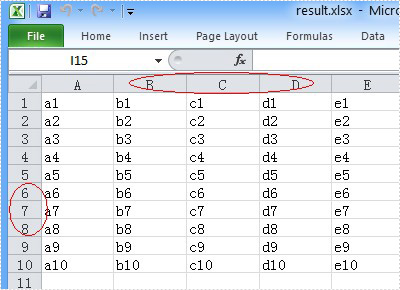Thursday, 23 January 2014 06:45

## Unhide the Excel Row and Column in C#

Rate this item

You can hide the Excel row or column by using the c# code, but a row or column also becomes hidden when you want to show the full Excel worksheet. You can unhide the Excel row and column by using the c# code. This article aims at introducing the method sheet.ShowRow and sheet.ShowColumn in the Excel .NET component Spire.Xls to show the hidden row and column.

First, let’s preview the hidden row and column.Here comes to the steps of the process.

Step 1: Create an instance of Spire.XLS.Workbook.

[C#]
```Workbook workbook = new Workbook();
```

Step 2: Load the existing Excel file that hidden the row and column in the specified path.

[C#]
```workbook.LoadFromFile("hide.xlsx");
```

Step 3: Get the first worksheet of the Excel file.

[C#]
```Worksheet sheet = workbook.Worksheets;
```

Step 4: Unhide the hidden row and column.

[C#]
```sheet.ShowRow(7);
sheet.ShowColumn(3);
```

Step 5: Generate the new Excel file.

[C#]
```workbook.SaveToFile("result.xlsx", ExcelVersion.Version2010);
```

Now let's preview the effect screenshot.Here is the full code.

[C#]
```static void Main(string[] args)
{
Workbook workbook = new Workbook();
Worksheet sheet = workbook.Worksheets;
// unhide the hidden row and column of the worksheet.
sheet.ShowRow(7);
sheet.ShowColumn(3);
workbook.SaveToFile("result.xlsx", ExcelVersion.Version2010);
}
```